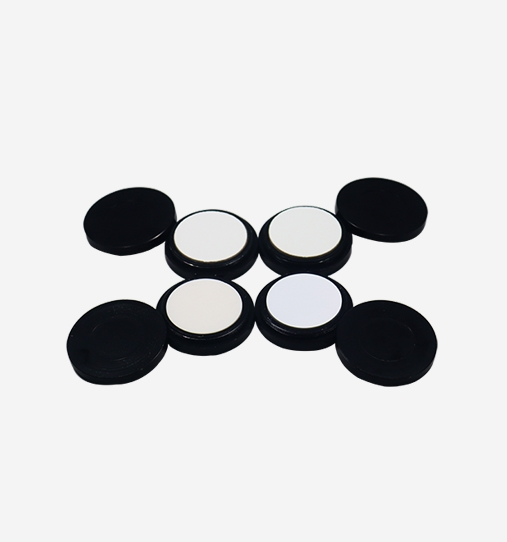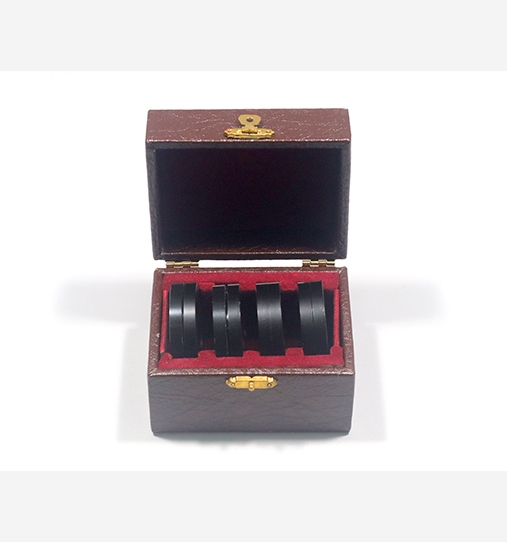﻿ 标准白板-备件、配件及零件-标准检测仪器制造商 | JIAYIINSTR
EN

PRODUCT CENTER98-01

R457白度值：100(高荧光板) 80(低荧光板) 80(工作白板1) 80(工作白板2)

1#工作白板:

D65/10º           X10=79.64      Y10= 84.43    Z10=90.21

x10= 0.3132   y10=0.3320

C/2º                X=82.37          Y=84.48        Z=99.50

x=0.3092       y=0.3172

R457=84.34

R950=77.55

2#工作白板:

D65/10º           X10=79.73      Y10= 84.54    Z10=90.29

x10= 0.3132   y10=0.3321

C/2º                 X=82.46         Y=84.59        Z=99.57

x=0.3093       y=0.3173

R457=84.41

R950=77.52

1#少量荧光板:

C-R457=76.39

D65-W86=77.45

D65-R457=83.35    C-W86=55.30    D65-Wcbg=68.78

2# 荧光板:

C-R457=90.75

D65-W86=127.01

D65-R457=100.02    C-W86=102.43    D65-Wcbg=177.50

*以上是某块该类型标准白板的测量数据，以此作为技术参数的范例。